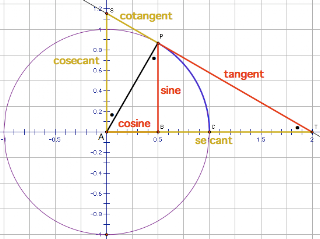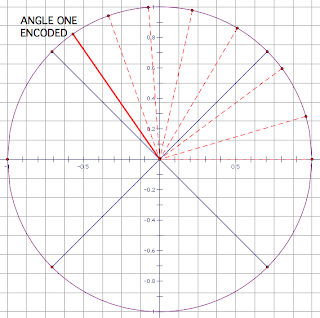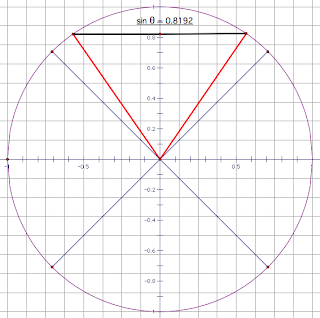## Tuesday, 30 July 2013

### MAT: TrigGate r=1

The unit circle can be used to estimate trigonometry, in place of a calculator. First, total credit for this idea goes to a colleague of mine, Esmeralda Fernandes. All I did was jazz it up, and present it as a Favourite idea at Twitter Math Camp 2013.

With that said, there is now a calculator that requires the user to enter a mental estimate; if the estimate is reasonable, you get the precise answer. It's the QAMA (Quick Approximate Mental Arithmetic) calculator. But even if you do not have one of those calculators, perhaps at some point you'll find yourself trapped on an alien world, with no power for your electronics, and to escape, you need to do some trigonometry. (Use your imagination.)

Fortunately, you can make yourself a unit circle:

Now, you've probably seen trig labelled in the unit circle before, but in a very generic way, as I've done below. I've even used that image myself, as part of my web serial, in Series 3. But what's with that generic triangle in Quadrant 1? Consider using a more specific triangle. One showing an actual angle.THIS IS TOO GENERIC

#### PRIMARY TRIGDO YOUR OWN SOUND EFFECTS
Pick an angle. Start dialing from (1, 0) - that is, spin your angle around the unit circle until you've located it's position. For instance, let's encode 125 degrees. (The 45 degree markers can help make an estimate, we know we need to be 10 degrees off of that.) Now simply drop perpendiculars to the axes! You can get a two decimal approximation, and check it on the calculator. Notice the sign of the trig comes right off of the axis, no need for that silly "CAST" rule.

Tangent is a little more interesting. Instead of actually drawing a tangent to the circle though, we can recognize it's merely slope. Rise over run. Sine over cosine. 0.82/-0.57, or simply -82/57. Which is somewhere around 1.5, in decimal. You don't even need to use the circle endpoint for this one, if you see it going through a grid square.CAN YOU SEE THE TANGENT HERE?

This can be done just as easily with radians; in fact the visual might make it easier. What's 5pi/6? Recognize pi is half the circle, and subdivide that into six parts. Now you've got your dialing point. Proceed exactly as above.

#### INVERSE TRIG

Time to work in reverse. Need to know what point to dial such that you get a result where sine is 0.8192? Sine: Start on the y-axis. Hopefully you can immediately see that there are two possible coordinates within 360 degrees. The first one is 55 degrees. Play around with the angles however you like to get that second measure of 125 - hopefully you can recognize that 90+55 is not a reasonable possibility.COSINE IS LEFT AS AN EXERCISE

Oh, you wanted radians? Well, the upper semicircle is already split into four equal parts. So we need a bit more than pi/4; how about pi/3? In other words, a bit more than one? (Ok, my calculator says pi/3.272, which is 0.96 or a bit less than one - but we're estimating here.) If I was really keen I could subdivide the half circle more accurately.

Oh, you were given sine as a negative? You're just working in the lower quadrants now. Oh, you were given tangent? That's dead easy! Rise by the decimal, and run by 1. Draw in the line. Or rise by half the amount and run by 0.5, if you're running out of space.

#### EXTENSIONS

Personally, I've only had the opportunity to show a class how it works, and they get a unit circle to use on their unit test. Possible other (better) extensions for you:
-Give them some of the basics (like sine), and see if they can extend (to figuring out tangent, for instance). Or to using the reciprocal ratios!
-Special angles also come into play, what do those triangles look like?
-You could turn this into a game, and see who can come up with the closest approximations.
-Even if you don't teach trig at all, you could use proportional reasoning to see how much of the circumference (~6.28) you cover if you only encode an angle of 80 degrees.

And one more Stargate connection: A Stargate is made up of 9 chevrons, each one equidistant from the others. The last chevron is positioned at the very top of the gate, 90 degrees. So what are the trig measures for the FIRST chevron, to it's right?SHOW WORK. ER, DON'T DRAW ON YOUR MONITOR